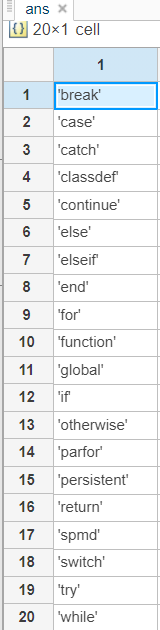Open in App
Not now

# Variable Names in MATLAB

• Difficulty Level : Easy
• Last Updated : 22 Sep, 2021

A variable is a named-memory location that stores different types of data which can be used to perform a specific set of operations. It can be thought of as a container that holds some value in memory. The Matlab workspace store all the variables being used during a session. This workspace not only deals with the creation of new variables but also supports the reusing of existing variables in the execution of the command. Each variable in the Matlab environment is treated as a matrix or an array of different data types. In Matlab, variables are assigned using the assignment ‘=’ operator.

Note:

• After the creation of a variable, we can use it later in our program.
• There must have values assigned to variables before they are used.
• If an expression returns a result without being assigned to any variable, the system implicitly assigns and stores the value to a special variable named ‘ans’. But ans variable is specific to the current workspace and the value of ans can change frequently, that is why the use of ans in a script or function is not recommended.

Example 1:

## Matlab

 `% MATLAB code for defining a and initializing  ` `% it with a value 10 using assignment operator '=' ` `a = 10`

Output:

`a = 10`

Example 2:

## Matlab

 `% MATLAB code for calculates an expression ` `% and stores value 13 in variable b ` `b = sqrt(169)`

Output:

`b = 13`

Example 3:

## Matlab

 `% MATLAB code to stores the expression  ` `% in special variable 'ans' ` `sqrt(169)`

Output:

`ans = 13`

## Rules for creating a variable:

A variable name is the name of the memory location where we can store our values. Some of the rules for naming a variable are:

• A variable name should start with a letter followed by digits, underscores, or letters.
• Don’t use Matlab keywords as variable names.
• Matlab is case sensitive, i.e., small letters and capital letters are treated differently. For example, variable names ‘PRICE’ and Price are different.
• A variable name cannot contain letters more than name length max characters.
• Special symbols anywhere in a variable name are syntactically invalid in Matlab.
• Avoid naming variable names as provided by pre-defined Matlab’s variable names.

## Check whether a variable name is aMatlab keyword:

To check whether a variable name is a MATLAB keyword or not, MATLAB provides a built-in function iskeyword( ). This keyword determines whether the input is MATLAB keyword or not.

Syntax:

v = iskeyword(txt)
iskeyword

Example 1:

## Matlab

 `% MATLAB code for check variable ` `% is keyword or not ` `Test=iskeyword(``'if'``)  ``% this statement returns  ` ` `  `% logical True (1) as a result as 'if' is a keyword  ` `% in the Matlab otherwise returns false.  ` ` `  `iskeyword ``if`  `% this statement uses the Matlab  ` `% command format and results in ans=1.             `

```Test = logical
1

ans = logical
1```

Example 2:

## Matlab

 `% MATLAB code for print all keyword ` `iskeyword ``% returns a list of all Matlab keywords.`

Output:## Check whether the input is a valid variable name:

To check whether the input is a valid variable name isvarname( ) is used. This function determines if the input is a valid variable name. If it is a valid MATLAB variable name the isvarname function returns logical 1 (true). Otherwise, it returns logical 0 (false).

Syntax:

t = isvarname(s)

isvarname s

Example 1:

## Matlab

 `% MATLAB code for isvarname ` `isvarname Number_1`

Output:

```ans = logical
1```

Example 2:

## Matlab

 `% MATLAB code for isvarname() ` `Test= isvarname(Digit_1)`

Output:

```Test = logical
1```

## Conflicts between variable and function name:

Matlab provides some predefined functions such as pi, ans, i, j, clock, date, eps which cannot be used as variable names as they can create confliction between variable name and functions name.  Usually, variable names take precedence over function names that result in unexpected results.

To check whether a variable name is already used with function:

Example:

## Matlab

 `% MATLAB code for check if there is no ` `% existing variables or functions name. In case it exists,  ` `% remove the variable from the memory with the clear() function.  ` `exist varname  `

Output:

`ans=0`

### genvarname()

This function (generate variable name)  is used to construct a valid and unique variable name. It returns an array of strings or characters that can be used as a legal variable name. Argument str can be a string, a string array, a character array, or a cell array containing character vectors. All the returned elements are unique.

Syntax:

varname = genvarname(str)

varname = genvarname(str, exclusions)

here genvarname(str, exclusions) returns a legal variable name that is different from any name listed in the exclusions input. The argument exclusions can be a string, a string array, a character array, a cell array of character vectors.

Example 1:

## Matlab

 `% MATLAB code for gemvarname() ` `var_name = genvarname({``'Pen'``, ``'Pen'``, ``'Pen'``, ``'Pen'``})`

Output:

```var_name = 1X4 cell
'Pen_1'   'Pen_2'   'Pen_3'    'Pen_4'```

Example 2:

## Matlab

 `% MATLAB code for genvarname() with different parameters ` `a = [``"Pen"``, ``"Eraser"``, ``"Pencil"``, ``"Box"` `] ` `var_name=genvarname(a,``"Box"``)`

Output:

```var_name= 1x4 string
"Pen"  "Eraser"   "Pencil"   "Box1"```

My Personal Notes arrow_drop_up
Related Articles